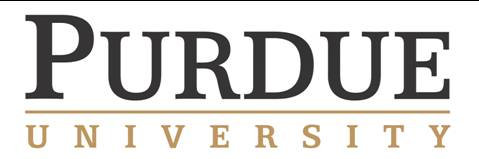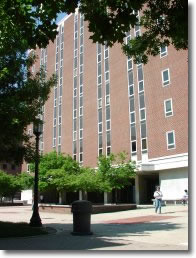Spring 2012 Midwest Topology Seminar
Abstracts## West Lafayette, INGunnar Carlson (Stanford):  Representations and K-theory
Recent years have seen striking developments in the calculation of the algebraic K-theory of fields. Specifically, there is a spectral sequence converging to the algebraic K-theory of a field $$F$$, whose $$E_2$$-term is describable in terms of cohomology groups of the absolute Galois group $$G_F$$. In this talk, we will describe a construction which constructs the K-theory spectrum of F itself from the representation theory of $$G_F$$ for a wide class of fields.  Byproducts are expressions of completed Milnor K-groups in terms of the homotopy groups of a derived version of completion of the representation ring of $$G_F$$.Fred Cohen
(U. of Rochester): Moment-angle complexes, Polyhedral products and their applications
This talk will give descriptions of the polyhedral product functor, and applications in joint work. with T. Bahri, M. Bendersky, S. Gitler. Further evolving applications with L. Taylor, F. Callegaro,
and M. Salvetti will also be given .

Dan Dugger (U. of Oregon):  Characteristic classes for Z/2-equivariant bundles
I will describe a recent computation of the $$RO(G)$$-graded, Eilenberg-MacLane cohomology of real, equivariant Grassmannians for the group $$G={\mathbf Z}/2$$.  The answer exhibits several interesting patterns related to the combinatorics of partitions, and the proof involves some  new techniques.  I will explain how this work ties in to some questions  about characteristic classes for quadratic bundles with values in mod 2 motivic cohomology.

Qayum Khan
(Notre Dame): Rigidity of pseudo-free group actions on contractible manifolds
We prove the following equivariant topological rigidity theorem.  Let $$X$$ be a contractible Riemannian $$n$$-manifold of nonpositive sectional curvature.  Let $$\Gamma$$ be a discrete cocompact group of isometries of $$X$$.  Suppose $$\Gamma$$ is virtually torsionfree and each nontrivial finite subgroup $$H$$ of $$\Gamma$$ has finite normalizer $$N_{\Gamma}(H)$$.
Assume $$n \geq 5$$.  Then there is a unique $$\Gamma$$-homeomorphism class of topological manifolds $$M$$ equipped with a cocompact proper $$\Gamma$$-action if $$\Gamma$$ is 2-torsionfree, or if $$n \equiv 0, 1 \pmod{4}$$.  For the other cases, where $$\Gamma$$ has elements of order two and $$n \equiv 2, 3 \pmod{4}$$, we show there are infinitely many such classes, and we completely classify them using quadratic forms and quadratic linking forms over the function field $$\mathbb{F}_2(t)$$.
The motivating special case comes from lifting the involutions on the $$n$$-torus $$T^n$$ that induce negative the identity map on the first integral homology group.

Matilde Marcolli (Caltech). Noncommutative numerical motives and the Tannakian formalism
I will describe recent work with Goncalo Tabuada, where we consider analogs of Grothendieck's standard conjectures C and D for a suitable category of noncommutative numerical motives and we show that, assuming these conjectures, one can make this category into a Tannakian category. The motivic Galois group of this category surjects onto the kernel of the homomorphism from the motivic Galois group of the category of (commutative) numerical motives to the multiplicative group, determined by the inclusion of the subcategory of Tate motives.

Lizhen Qin
(Purdue):  A Bona Fide CW Decomposition of Compact Manifolds Resulting from Morse Theory
Consider a Morse function on a compact Riemannian manifold. This manifold is a disjoint union of unstable manifolds of the negative gradient of the Morse function. These unstable manifolds are open cells. Does this give a CW complex which is homeomorphic (not only homotopy equivalent) to the compact manifold? This talk will describe the history of the above problem and give an affirmative answer to it.

Sasha Voronov (U. of Minnesota): A higher category of cobordisms and TQFT
I will describe a categorical formalism for (Extended) Topological Quantum Field Theories (TQFTs) and present them as functors from a suitable category of cobordisms with corners to a (linear) category, generalizing open-closed string theory to higher dimensions. The approach is based on the notion of an n-fold category by C. Ehresmann, weakened in the spirit of monoidal categories (associators, interchangers, Mac Lane's pentagons and hexagons), in contrast with the simplicial (weak Kan and complete Segal) approach of Jacob Lurie.